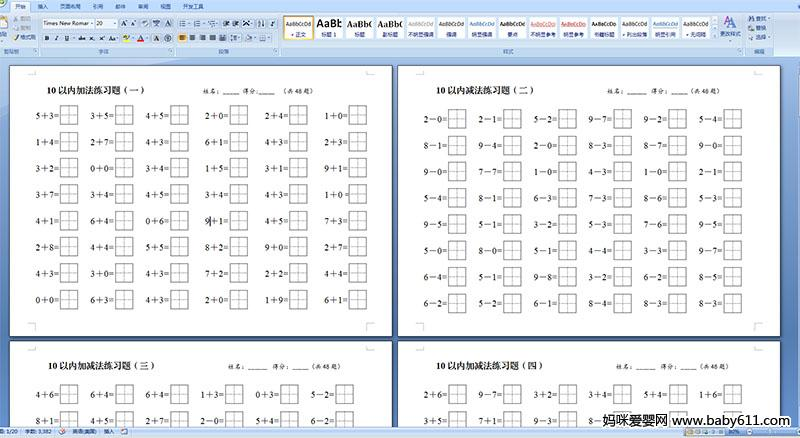# гзЖљдА10вдФкМгЗЈСЗЯАЬт wordАцБО

РрБ№ЃКЪ§бЇЪдОэЁЁИќаТЪБМфЃК2018Фъ04дТ12Ше 22:50:08

WORDИёЪНЃЌЯТдиКѓПЩжБНгДђгЁЃЌвЛЙВ20вГЃЌЕуЛїЯТЗНЭМЦЌЯТдиЁЁЁЁ10вдФкМгЗЈСЗЯАЬтЃЈвЛЃЉ                 аеУћЃК_____  ЕУЗж:_____  ЃЈЙВ48ЬтЃЉ5ЃЋ3=       3ЃЋ5=       4ЃЋ5=        2ЃЋ0=        2ЃЋ4=        1ЃЋ0=1ЃЋ4=       2ЃЋ7=       4ЃЋ3=        6ЃЋ1=        4ЃЋ3=        2ЃЋ3=3ЃЋ2=        0ЃЋ0=       3ЃЋ4=        1ЃЋ5=        3ЃЋ1=        9ЃЋ1=3ЃЋ7=       3ЃЋ4=       4ЃЋ5=        3ЃЋ4=        4ЃЋ3=        1ЃЋ0 =4ЃЋ1=       6ЃЋ4=       0ЃЋ6=        9ЃЋ1=        4ЃЋ5=        7ЃЋ3=2ЃЋ8=       4ЃЋ4=       5ЃЋ5=        8ЃЋ2=        9ЃЋ0=        2ЃЋ7=4ЃЋ3=       3ЃЋ0=       4ЃЋ3=        7ЃЋ2=        2ЃЋ2=        4ЃЋ4=0ЃЋ0=       6ЃЋ3=       4ЃЋ3=        2ЃЋ0=        1ЃЋ9=        6ЃЋ1=10вдФкМѕЗЈСЗЯАЬтЃЈЖўЃЉ               аеУћЃК______ ЕУЗжЃК_____ЃЈЙВ48ЬтЃЉ2Ѓ­0=       2Ѓ­1=       5Ѓ­2=       9Ѓ­7=       9Ѓ­2=       5Ѓ­4=8Ѓ­1=       9Ѓ­4=       2Ѓ­0=          8Ѓ­3=          8Ѓ­7=       9Ѓ­0=9Ѓ­0=       7Ѓ­7=       1Ѓ­0=       4Ѓ­3=       1Ѓ­0=       2Ѓ­1=5Ѓ­4=         8Ѓ­1=       6Ѓ­3=       7Ѓ­3=       8Ѓ­6=       5Ѓ­3=9Ѓ­5=       5Ѓ­1=       3Ѓ­2=       5Ѓ­3=       7Ѓ­6=       9Ѓ­5=5Ѓ­0=         8Ѓ­0=         5Ѓ­1=       4Ѓ­4=       3Ѓ­3=       9Ѓ­7=6Ѓ­4=       5Ѓ­1=       9Ѓ­8=       3Ѓ­2=       6Ѓ­2=       8Ѓ­5=6Ѓ­2=       5Ѓ­2=       6Ѓ­1=       8Ѓ­4=       8Ѓ­3=       8Ѓ­3=10вдФкМгМѕЗЈСЗЯАЬтЃЈШ§ЃЉ               аеУћЃК______ ЕУЗжЃК_____ЃЈЙВ48ЬтЃЉ4ЃЋ6=       6ЃЋ4=       6ЃЋ4=       1ЃЋ3=       0ЃЋ3=       5Ѓ­2=8ЃЋ1=       4ЃЋ5=       2ЃЋ7=       2Ѓ­0=       5ЃЋ4=       6Ѓ­2=3ЃЋ4=       1Ѓ­1=       4Ѓ­2=       1Ѓ­1=       5ЃЋ0=       7Ѓ­3=4ЃЋ3=       4ЃЋ4=       6Ѓ­5=       4ЃЋ4=       4ЃЋ4=       4Ѓ­0=5Ѓ­2=       0ЃЋ7=       7ЃЋ1=       2ЃЋ0=       9Ѓ­6=       3Ѓ­1=9Ѓ­3=       5ЃЋ4=       6Ѓ­6=       3ЃЋ4=       1ЃЋ0=       6ЃЋ2=9Ѓ­4=       1ЃЋ4=       4Ѓ­0=       1ЃЋ8=       2ЃЋ3=       4ЃЋ2=4ЃЋ3=       4Ѓ­0=       1Ѓ­1=       3ЃЋ4=       2ЃЋ8=       7Ѓ­2=10вдФкМгМѕЗЈСЗЯАЬтЃЈЫФЃЉ               аеУћЃК______ ЕУЗжЃК_____ЃЈЙВ48ЬтЃЉ2ЃЋ6=       9Ѓ­7=       3ЃЋ2=       3ЃЋ4=       5ЃЋ4=       1ЃЋ6=3ЃЋ5=       7ЃЋ1=       9Ѓ­3=       8Ѓ­3=       5Ѓ­4=       4Ѓ­0=8Ѓ­2=       7Ѓ­2=       6Ѓ­4=       9Ѓ­2=      10Ѓ­9=       1ЃЋ1=5ЃЋ5=       5ЃЋ2=       9Ѓ­4=       8ЃЋ1=       2Ѓ­1=       8ЃЋ1=4ЃЋ6=       2ЃЋ7=       9Ѓ­5=       3ЃЋ3=       4Ѓ­2=       4Ѓ­1=1ЃЋ2=      10Ѓ­4=       5Ѓ­3=       0ЃЋ8=      10Ѓ­7=       9Ѓ­3=2ЃЋ4=       6Ѓ­5=       4ЃЋ4=       5Ѓ­1=       1ЃЋ9=       3ЃЋ6=8Ѓ­4=       6ЃЋ0=       3Ѓ­3=       1ЃЋ5=      10Ѓ­5=       3ЃЋ2=10вдФкМгМѕЗЈСЗЯАЬтЃЈЮхЃЉ               аеУћЃК______ ЕУЗжЃК_____ЃЈЙВ48ЬтЃЉ2ЃЋ6=       9Ѓ­7=       3ЃЋ2=       3ЃЋ4=       5ЃЋ4=       1ЃЋ6=3ЃЋ5=       7ЃЋ1=       9Ѓ­3=       8Ѓ­3=       5Ѓ­4=       4Ѓ­0=8Ѓ­2=       7Ѓ­2=       6Ѓ­4=       9Ѓ­2=      10Ѓ­9=       1ЃЋ1=5ЃЋ5=       5ЃЋ2=       9Ѓ­4=       8ЃЋ1=       2Ѓ­1=       8ЃЋ1=4ЃЋ6=       2ЃЋ7=       9Ѓ­5=       3ЃЋ3=       4Ѓ­2=       4Ѓ­1=1ЃЋ2=      10Ѓ­4=       5Ѓ­3=       0ЃЋ8=      10Ѓ­7=       9Ѓ­3=2ЃЋ4=       6Ѓ­5=       4ЃЋ4=       5Ѓ­1=       1ЃЋ9=       3ЃЋ6=8Ѓ­4=       6ЃЋ0=       3Ѓ­3=       1ЃЋ5=      10Ѓ­5=       3ЃЋ2=10вдФкМгМѕЗЈСЗЯАЬтЃЈСљЃЉ               аеУћЃК______ ЕУЗжЃК_____ЃЈЙВ48ЬтЃЉ4ЃЋ6=       6ЃЋ4=       6ЃЋ4=       1ЃЋ3=       0ЃЋ3=       5Ѓ­2=8ЃЋ1=       4ЃЋ5=       2ЃЋ7=       2Ѓ­0=       5ЃЋ4=       6Ѓ­2=3ЃЋ4=       1Ѓ­1=       4Ѓ­2=       1Ѓ­1=       5ЃЋ0=       7Ѓ­3=4ЃЋ3=       4ЃЋ4=       6Ѓ­5=       4ЃЋ4=       4ЃЋ4=       4Ѓ­0=5Ѓ­2=       0ЃЋ7=       7ЃЋ1=       2ЃЋ0=       9Ѓ­6=       3Ѓ­1=9Ѓ­3=       5ЃЋ4=       6Ѓ­6=       3ЃЋ4=       1ЃЋ0=       6ЃЋ2=9Ѓ­4=       1ЃЋ4=       4Ѓ­0=       1ЃЋ8=       2ЃЋ3=       4ЃЋ2=4ЃЋ3=       4Ѓ­0=       1Ѓ­1=       3ЃЋ4=       2ЃЋ8=       7Ѓ­2=10вдФкМгЗЈСЗЯАЬтЃЈЦпЃЉ                 аеУћЃК_____  ЕУЗж:_____  ЃЈЙВ48ЬтЃЉ5ЃЋ3=       3ЃЋ5=       4ЃЋ5=        2ЃЋ0=        2ЃЋ4=        1ЃЋ0=1ЃЋ4=       2ЃЋ7=       4ЃЋ3=        6ЃЋ1=        4ЃЋ3=        2ЃЋ3=3ЃЋ2=        0ЃЋ0=       3ЃЋ4=        1ЃЋ5=        3ЃЋ1=        9ЃЋ1=3ЃЋ7=       3ЃЋ4=       4ЃЋ5=        3ЃЋ4=        4ЃЋ3=        1ЃЋ0 =4ЃЋ1=       6ЃЋ4=       0ЃЋ6=        9ЃЋ1=        4ЃЋ5=        7ЃЋ3=2ЃЋ8=       4ЃЋ4=       5ЃЋ5=        8ЃЋ2=        9ЃЋ0=        2ЃЋ7=4ЃЋ3=       3ЃЋ0=       4ЃЋ3=        7ЃЋ2=        2ЃЋ2=        4ЃЋ4=0ЃЋ0=       6ЃЋ3=       4ЃЋ3=        2ЃЋ0=        1ЃЋ9=        6ЃЋ1=10вдФкМѕЗЈСЗЯАЬтЃЈАЫЃЉ               аеУћЃК______ ЕУЗжЃК_____ЃЈЙВ48ЬтЃЉ2Ѓ­0=       2Ѓ­1=       5Ѓ­2=       9Ѓ­7=       9Ѓ­2=       5Ѓ­4=8Ѓ­1=       9Ѓ­4=       2Ѓ­0=          8Ѓ­3=          8Ѓ­7=       9Ѓ­0=9Ѓ­0=       7Ѓ­7=       1Ѓ­0=       4Ѓ­3=       1Ѓ­0=       2Ѓ­1=5Ѓ­4=         8Ѓ­1=       6Ѓ­3=       7Ѓ­3=       8Ѓ­6=       5Ѓ­3=9Ѓ­5=       5Ѓ­1=       3Ѓ­2=       5Ѓ­3=       7Ѓ­6=       9Ѓ­5=5Ѓ­0=         8Ѓ­0=         5Ѓ­1=       4Ѓ­4=       3Ѓ­3=       9Ѓ­7=6Ѓ­4=       5Ѓ­1=       9Ѓ­8=       3Ѓ­2=       6Ѓ­2=       8Ѓ­5=6Ѓ­2=       5Ѓ­2=       6Ѓ­1=       8Ѓ­4=       8Ѓ­3=       8Ѓ­3=10вдФкМгМѕЗЈСЗЯАЬтЃЈОХЃЉ               аеУћЃК______ ЕУЗжЃК_____ЃЈЙВ48ЬтЃЉ4ЃЋ6=       6ЃЋ4=       6ЃЋ4=       1ЃЋ3=       0ЃЋ3=       5Ѓ­2=8ЃЋ1=       4ЃЋ5=       2ЃЋ7=       2Ѓ­0=       5ЃЋ4=       6Ѓ­2=3ЃЋ4=       1Ѓ­1=       4Ѓ­2=       1Ѓ­1=       5ЃЋ0=       7Ѓ­3=4ЃЋ3=       4ЃЋ4=       6Ѓ­5=       4ЃЋ4=       4ЃЋ4=       4Ѓ­0=5Ѓ­2=       0ЃЋ7=       7ЃЋ1=       2ЃЋ0=       9Ѓ­6=       3Ѓ­1=9Ѓ­3=       5ЃЋ4=       6Ѓ­6=       3ЃЋ4=       1ЃЋ0=       6ЃЋ2=9Ѓ­4=       1ЃЋ4=       4Ѓ­0=       1ЃЋ8=       2ЃЋ3=       4ЃЋ2=4ЃЋ3=       4Ѓ­0=       1Ѓ­1=       3ЃЋ4=       2ЃЋ8=       7Ѓ­2=10вдФкМгМѕЗЈСЗЯАЬтЃЈЪЎЃЉ               аеУћЃК______ ЕУЗжЃК_____ЃЈЙВ48ЬтЃЉ2ЃЋ6=       9Ѓ­7=       3ЃЋ2=       3ЃЋ4=       5ЃЋ4=       1ЃЋ6=3ЃЋ5=       7ЃЋ1=       9Ѓ­3=       8Ѓ­3=       5Ѓ­4=       4Ѓ­0=8Ѓ­2=       7Ѓ­2=       6Ѓ­4=       9Ѓ­2=      10Ѓ­9=       1ЃЋ1=5ЃЋ5=       5ЃЋ2=       9Ѓ­4=       8ЃЋ1=       2Ѓ­1=       8ЃЋ1=4ЃЋ6=       2ЃЋ7=       9Ѓ­5=       3ЃЋ3=       4Ѓ­2=       4Ѓ­1=1ЃЋ2=      10Ѓ­4=       5Ѓ­3=       0ЃЋ8=      10Ѓ­7=       9Ѓ­3=2ЃЋ4=       6Ѓ­5=       4ЃЋ4=       5Ѓ­1=       1ЃЋ9=       3ЃЋ6=8Ѓ­4=       6ЃЋ0=       3Ѓ­3=       1ЃЋ5=      10Ѓ­5=       3ЃЋ2=10вдФкМгЗЈСЗЯАЬтЃЈЪЎвЛЃЉ                 аеУћЃК_____  ЕУЗж:_____  ЃЈЙВ48ЬтЃЉ5ЃЋ3=       3ЃЋ5=       4ЃЋ5=        2ЃЋ0=        2ЃЋ4=        1ЃЋ0=1ЃЋ4=       2ЃЋ7=       4ЃЋ3=        6ЃЋ1=        4ЃЋ3=        2ЃЋ3=3ЃЋ2=        0ЃЋ0=       3ЃЋ4=        1ЃЋ5=        3ЃЋ1=        9ЃЋ1=3ЃЋ7=       3ЃЋ4=       4ЃЋ5=        3ЃЋ4=        4ЃЋ3=        1ЃЋ0 =4ЃЋ1=       6ЃЋ4=       0ЃЋ6=        9ЃЋ1=        4ЃЋ5=        7ЃЋ3=2ЃЋ8=       4ЃЋ4=       5ЃЋ5=        8ЃЋ2=        9ЃЋ0=        2ЃЋ7=4ЃЋ3=       3ЃЋ0=       4ЃЋ3=        7ЃЋ2=        2ЃЋ2=        4ЃЋ4=0ЃЋ0=       6ЃЋ3=       4ЃЋ3=        2ЃЋ0=        1ЃЋ9=        6ЃЋ1=10вдФкМѕЗЈСЗЯАЬтЃЈЪЎЖўЃЉ               аеУћЃК______ ЕУЗжЃК_____ЃЈЙВ48ЬтЃЉ2Ѓ­0=       2Ѓ­1=       5Ѓ­2=       9Ѓ­7=       9Ѓ­2=       5Ѓ­4=8Ѓ­1=       9Ѓ­4=       2Ѓ­0=          8Ѓ­3=          8Ѓ­7=       9Ѓ­0=9Ѓ­0=       7Ѓ­7=       1Ѓ­0=       4Ѓ­3=       1Ѓ­0=       2Ѓ­1=5Ѓ­4=         8Ѓ­1=       6Ѓ­3=       7Ѓ­3=       8Ѓ­6=       5Ѓ­3=9Ѓ­5=       5Ѓ­1=       3Ѓ­2=       5Ѓ­3=       7Ѓ­6=       9Ѓ­5=5Ѓ­0=         8Ѓ­0=         5Ѓ­1=       4Ѓ­4=       3Ѓ­3=       9Ѓ­7=6Ѓ­4=       5Ѓ­1=       9Ѓ­8=       3Ѓ­2=       6Ѓ­2=       8Ѓ­5=6Ѓ­2=       5Ѓ­2=       6Ѓ­1=       8Ѓ­4=       8Ѓ­3=       8Ѓ­3=10вдФкМгМѕЗЈСЗЯАЬтЃЈЪЎШ§ЃЉ               аеУћЃК______ ЕУЗжЃК_____ЃЈЙВ48ЬтЃЉ4ЃЋ6=       6ЃЋ4=       6ЃЋ4=       1ЃЋ3=       0ЃЋ3=       5Ѓ­2=8ЃЋ1=       4ЃЋ5=       2ЃЋ7=       2Ѓ­0=       5ЃЋ4=       6Ѓ­2=3ЃЋ4=       1Ѓ­1=       4Ѓ­2=       1Ѓ­1=       5ЃЋ0=       7Ѓ­3=4ЃЋ3=       4ЃЋ4=       6Ѓ­5=       4ЃЋ4=       4ЃЋ4=       4Ѓ­0=5Ѓ­2=       0ЃЋ7=       7ЃЋ1=       2ЃЋ0=       9Ѓ­6=       3Ѓ­1=9Ѓ­3=       5ЃЋ4=       6Ѓ­6=       3ЃЋ4=       1ЃЋ0=       6ЃЋ2=9Ѓ­4=       1ЃЋ4=       4Ѓ­0=       1ЃЋ8=       2ЃЋ3=       4ЃЋ2=4ЃЋ3=       4Ѓ­0=       1Ѓ­1=       3ЃЋ4=       2ЃЋ8=       7Ѓ­2=10вдФкМгМѕЗЈСЗЯАЬтЃЈЪЎЫФЃЉ               аеУћЃК______ ЕУЗжЃК_____ЃЈЙВ48ЬтЃЉ2ЃЋ6=       9Ѓ­7=       3ЃЋ2=       3ЃЋ4=       5ЃЋ4=       1ЃЋ6=3ЃЋ5=       7ЃЋ1=       9Ѓ­3=       8Ѓ­3=       5Ѓ­4=       4Ѓ­0=8Ѓ­2=       7Ѓ­2=       6Ѓ­4=       9Ѓ­2=      10Ѓ­9=       1ЃЋ1=5ЃЋ5=       5ЃЋ2=       9Ѓ­4=       8ЃЋ1=       2Ѓ­1=       8ЃЋ1=4ЃЋ6=       2ЃЋ7=       9Ѓ­5=       3ЃЋ3=       4Ѓ­2=       4Ѓ­1=1ЃЋ2=      10Ѓ­4=       5Ѓ­3=       0ЃЋ8=      10Ѓ­7=       9Ѓ­3=2ЃЋ4=       6Ѓ­5=       4ЃЋ4=       5Ѓ­1=       1ЃЋ9=       3ЃЋ6=8Ѓ­4=       6ЃЋ0=       3Ѓ­3=       1ЃЋ5=      10Ѓ­5=       3ЃЋ2=10вдФкМгМѕЗЈСЗЯАЬтЃЈЪЎЮхЃЉ               аеУћЃК______ ЕУЗжЃК_____ЃЈЙВ48ЬтЃЉ2ЃЋ6=       9Ѓ­7=       3ЃЋ2=       3ЃЋ4=       5ЃЋ4=       1ЃЋ6=3ЃЋ5=       7ЃЋ1=       9Ѓ­3=       8Ѓ­3=       5Ѓ­4=       4Ѓ­0=8Ѓ­2=       7Ѓ­2=       6Ѓ­4=       9Ѓ­2=      10Ѓ­9=       1ЃЋ1=5ЃЋ5=       5ЃЋ2=       9Ѓ­4=       8ЃЋ1=       2Ѓ­1=       8ЃЋ1=4ЃЋ6=       2ЃЋ7=       9Ѓ­5=       3ЃЋ3=       4Ѓ­2=       4Ѓ­1=1ЃЋ2=      10Ѓ­4=       5Ѓ­3=       0ЃЋ8=      10Ѓ­7=       9Ѓ­3=2ЃЋ4=       6Ѓ­5=       4ЃЋ4=       5Ѓ­1=       1ЃЋ9=       3ЃЋ6=8Ѓ­4=       6ЃЋ0=       3Ѓ­3=       1ЃЋ5=      10Ѓ­5=       3ЃЋ2=10вдФкМгМѕЗЈСЗЯАЬтЃЈЪЎСљЃЉ               аеУћЃК______ ЕУЗжЃК_____ЃЈЙВ48ЬтЃЉ4ЃЋ6=       6ЃЋ4=       6ЃЋ4=       1ЃЋ3=       0ЃЋ3=       5Ѓ­2=8ЃЋ1=       4ЃЋ5=       2ЃЋ7=       2Ѓ­0=       5ЃЋ4=       6Ѓ­2=3ЃЋ4=       1Ѓ­1=       4Ѓ­2=       1Ѓ­1=       5ЃЋ0=       7Ѓ­3=4ЃЋ3=       4ЃЋ4=       6Ѓ­5=       4ЃЋ4=       4ЃЋ4=       4Ѓ­0=5Ѓ­2=       0ЃЋ7=       7ЃЋ1=       2ЃЋ0=       9Ѓ­6=       3Ѓ­1=9Ѓ­3=       5ЃЋ4=       6Ѓ­6=       3ЃЋ4=       1ЃЋ0=       6ЃЋ2=9Ѓ­4=       1ЃЋ4=       4Ѓ­0=       1ЃЋ8=       2ЃЋ3=       4ЃЋ2=4ЃЋ3=       4Ѓ­0=       1Ѓ­1=       3ЃЋ4=       2ЃЋ8=       7Ѓ­2=10вдФкМгЗЈСЗЯАЬтЃЈЪЎЦпЃЉ                 аеУћЃК_____  ЕУЗж:_____  ЃЈЙВ48ЬтЃЉ5ЃЋ3=       3ЃЋ5=       4ЃЋ5=        2ЃЋ0=        2ЃЋ4=        1ЃЋ0=1ЃЋ4=       2ЃЋ7=       4ЃЋ3=        6ЃЋ1=        4ЃЋ3=        2ЃЋ3=3ЃЋ2=        0ЃЋ0=       3ЃЋ4=        1ЃЋ5=        3ЃЋ1=        9ЃЋ1=3ЃЋ7=       3ЃЋ4=       4ЃЋ5=        3ЃЋ4=        4ЃЋ3=        1ЃЋ0 =4ЃЋ1=       6ЃЋ4=       0ЃЋ6=        9ЃЋ1=        4ЃЋ5=        7ЃЋ3=2ЃЋ8=       4ЃЋ4=       5ЃЋ5=        8ЃЋ2=        9ЃЋ0=        2ЃЋ7=4ЃЋ3=       3ЃЋ0=       4ЃЋ3=        7ЃЋ2=        2ЃЋ2=        4ЃЋ4=0ЃЋ0=       6ЃЋ3=       4ЃЋ3=        2ЃЋ0=        1ЃЋ9=        6ЃЋ1=10вдФкМѕЗЈСЗЯАЬтЃЈЪЎАЫЃЉ               аеУћЃК______ ЕУЗжЃК_____ЃЈЙВ48ЬтЃЉ2Ѓ­0=       2Ѓ­1=       5Ѓ­2=       9Ѓ­7=       9Ѓ­2=       5Ѓ­4=8Ѓ­1=       9Ѓ­4=       2Ѓ­0=          8Ѓ­3=          8Ѓ­7=       9Ѓ­0=9Ѓ­0=       7Ѓ­7=       1Ѓ­0=       4Ѓ­3=       1Ѓ­0=       2Ѓ­1=5Ѓ­4=         8Ѓ­1=       6Ѓ­3=       7Ѓ­3=       8Ѓ­6=       5Ѓ­3=9Ѓ­5=       5Ѓ­1=       3Ѓ­2=       5Ѓ­3=       7Ѓ­6=       9Ѓ­5=5Ѓ­0=         8Ѓ­0=         5Ѓ­1=       4Ѓ­4=       3Ѓ­3=       9Ѓ­7=6Ѓ­4=       5Ѓ­1=       9Ѓ­8=       3Ѓ­2=       6Ѓ­2=       8Ѓ­5=6Ѓ­2=       5Ѓ­2=       6Ѓ­1=       8Ѓ­4=       8Ѓ­3=       8Ѓ­3=10вдФкМгМѕЗЈСЗЯАЬтЃЈЪЎОХЃЉ               аеУћЃК______ ЕУЗжЃК_____ЃЈЙВ48ЬтЃЉ4ЃЋ6=       6ЃЋ4=       6ЃЋ4=       1ЃЋ3=       0ЃЋ3=       5Ѓ­2=8ЃЋ1=       4ЃЋ5=       2ЃЋ7=       2Ѓ­0=       5ЃЋ4=       6Ѓ­2=3ЃЋ4=       1Ѓ­1=       4Ѓ­2=       1Ѓ­1=       5ЃЋ0=       7Ѓ­3=4ЃЋ3=       4ЃЋ4=       6Ѓ­5=       4ЃЋ4=       4ЃЋ4=       4Ѓ­0=5Ѓ­2=       0ЃЋ7=       7ЃЋ1=       2ЃЋ0=       9Ѓ­6=       3Ѓ­1=9Ѓ­3=       5ЃЋ4=       6Ѓ­6=       3ЃЋ4=       1ЃЋ0=       6ЃЋ2=9Ѓ­4=       1ЃЋ4=       4Ѓ­0=       1ЃЋ8=       2ЃЋ3=       4ЃЋ2=4ЃЋ3=       4Ѓ­0=       1Ѓ­1=       3ЃЋ4=       2ЃЋ8=       7Ѓ­2=10вдФкМгМѕЗЈСЗЯАЬтЃЈЖўЪЎЃЉ               аеУћЃК______ ЕУЗжЃК_____ЃЈЙВ48ЬтЃЉ2ЃЋ6=       9Ѓ­7=       3ЃЋ2=       3ЃЋ4=       5ЃЋ4=       1ЃЋ6=3ЃЋ5=       7ЃЋ1=       9Ѓ­3=       8Ѓ­3=       5Ѓ­4=       4Ѓ­0=8Ѓ­2=       7Ѓ­2=       6Ѓ­4=       9Ѓ­2=      10Ѓ­9=       1ЃЋ1=5ЃЋ5=       5ЃЋ2=       9Ѓ­4=       8ЃЋ1=       2Ѓ­1=       8ЃЋ1=4ЃЋ6=       2ЃЋ7=       9Ѓ­5=       3ЃЋ3=       4Ѓ­2=       4Ѓ­1=1ЃЋ2=      10Ѓ­4=       5Ѓ­3=       0ЃЋ8=      10Ѓ­7=       9Ѓ­3=2ЃЋ4=       6Ѓ­5=       4ЃЋ4=       5Ѓ­1=       1ЃЋ9=       3ЃЋ6=8Ѓ­4=       6ЃЋ0=       3Ѓ­3=       1ЃЋ5=      10Ѓ­5=       3ЃЋ2=

ЬсЪОЃКЩЯЭМЮЊИУЪдОэЕФWORDНиЭМЃЌЯТдиКѓПЩжБНгДђгЁЃЌЧыЕуЛїЯТЗНАДХЅЯТдиИУЪдОэ
Ъ§бЇЪдОэЯрЙи
• гзЖљдАЪдОэЗжРр
• НЋДЫЪдОэЗжЯэЕНЃК
• Ъ§бЇЪдОэзюаТИќаТ